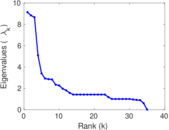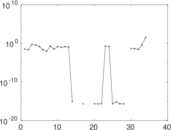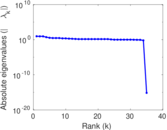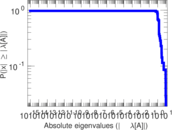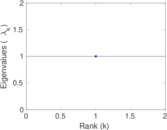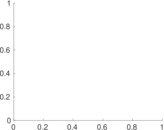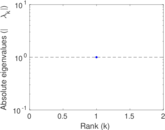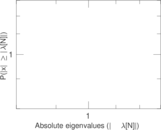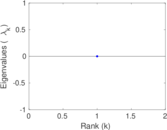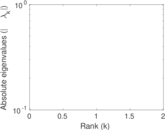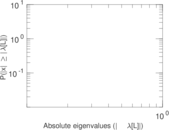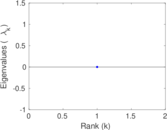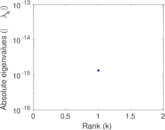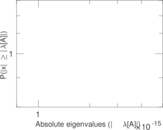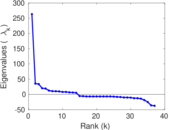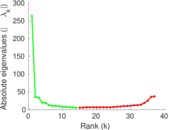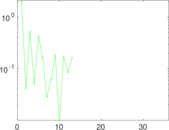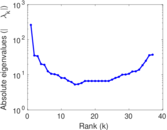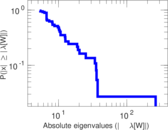# Wiktionary edits (sn)

This is the bipartite edit network of the Shona Wiktionary. It contains users and pages from the Shona Wiktionary, connected by edit events. Each edge represents an edit. The dataset includes the timestamp of each edit.

 Code `msn` Internal name `edit-snwiktionary` Name Wiktionary edits (sn) Data source http://dumps.wikimedia.org/ AvailabilityDataset is available for download Consistency checkDataset passed all tests Category Authorship network Dataset timestamp 2017-10-20 Node meaning User, article Edge meaning Edit Network formatBipartite, undirected Edge typeUnweighted, multiple edges Temporal dataEdges are annotated with timestamps

## Statistics

 Size n = 270 Left size n1 = 37 Right size n2 = 233 Volume m = 295 Unique edge count m̿ = 271 Wedge count s = 6,255 Claw count z = 146,005 Cross count x = 2,651,049 Square count q = 124 4-Tour count T4 = 26,862 Maximum degree dmax = 78 Maximum left degree d1max = 78 Maximum right degree d2max = 13 Average degree d = 2.185 19 Average left degree d1 = 7.972 97 Average right degree d2 = 1.266 09 Fill p = 0.031 434 9 Average edge multiplicity m̃ = 1.088 56 Size of LCC N = 78 Diameter δ = 2 50-Percentile effective diameter δ0.5 = 1.481 26 90-Percentile effective diameter δ0.9 = 1.896 25 Median distance δM = 2 Mean distance δm = 1.952 09 Gini coefficient G = 0.548 992 Balanced inequality ratio P = 0.281 356 Left balanced inequality ratio P1 = 0.196 610 Right balanced inequality ratio P2 = 0.433 898 Relative edge distribution entropy Her = 0.817 383 Power law exponent γ = 5.547 10 Tail power law exponent γt = 2.731 00 Tail power law exponent with p γ3 = 2.731 00 p-value p = 0.075 000 0 Left tail power law exponent with p γ3,1 = 1.941 00 Left p-value p1 = 0.637 000 Right tail power law exponent with p γ3,2 = 3.531 00 Right p-value p2 = 0.026 000 0 Degree assortativity ρ = −0.384 464 Degree assortativity p-value pρ = 5.623 37 × 10−11 Spectral norm α = 9.131 94 Spectral separation |λ1[A] / λ2[A]| = 1.033 99 Controllability C = 200 Relative controllability Cr = 0.746 269

## Plots

### Fruchterman–Reingold graph drawing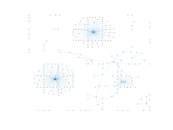### Degree distribution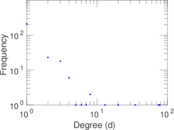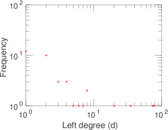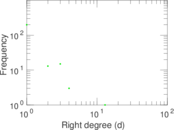### Cumulative degree distribution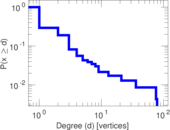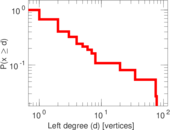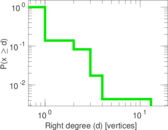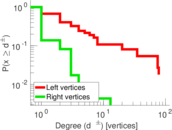### Lorenz curve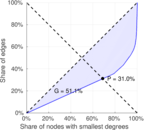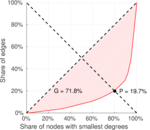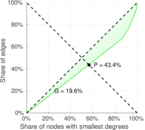### Spectral distribution of the adjacency matrix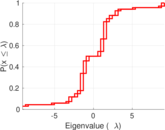### Spectral distribution of the normalized adjacency matrix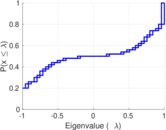### Spectral distribution of the Laplacian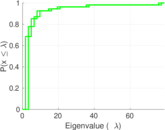### Spectral graph drawing based on the adjacency matrix### Spectral graph drawing based on the normalized adjacency matrix### Degree assortativity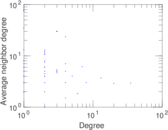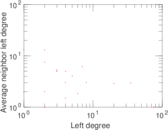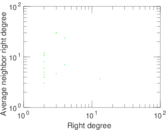### Zipf plot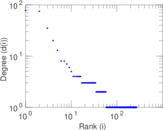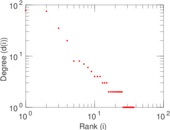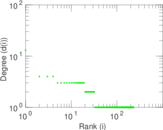### Hop distribution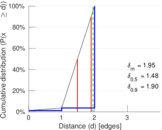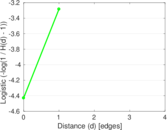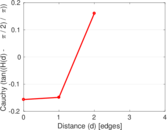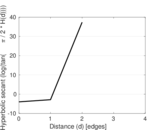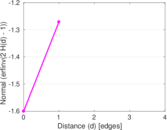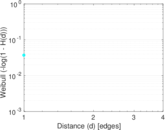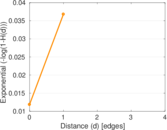### Double Laplacian graph drawing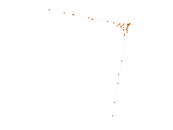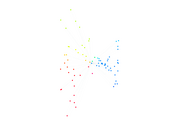### Delaunay graph drawing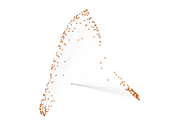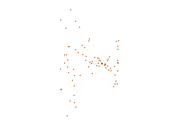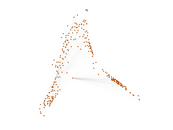### Edge weight/multiplicity distribution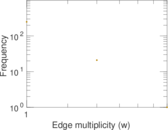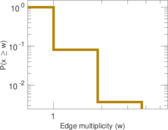### Temporal distribution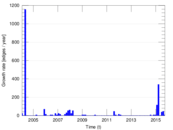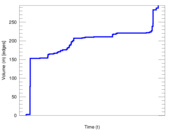### Temporal hop distribution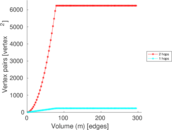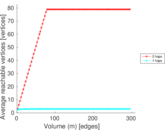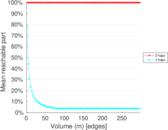### Diameter/density evolution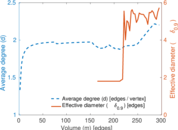### Matrix decompositions plots